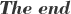2018-07-13 13:52:25 eaglesimu 阅读数 177
• ###### 系统集成项目管理工程师考试-项目管理部分大全

该部分为考试重要的部分所在，上午选择题40分左右，下午案例分析甚至占到百分之90，需要花大的精力去掌握，去融会贯通，44个过程、129个工具、每个活动的内容必须掌握，当然我们在串讲的时候运用了多种个性化的方法，输入输出大家觉得很难，这部分选择性掌握。

12018 人正在学习 去看看 赵冲

### Part i 算法原理

=============================朴素贝叶斯=============================

# 输出：某个样本的分类

Step.1 计算先验概率和条件概率Step.2 对于给定样本，计算该样本属于各类的概率Step.3 确定样本属于哪一类

#1. 在计算某个条件概率时如果出现了0的情况，则很有可能会影响到厚颜概率的计算。
2. 对于离散变量可以很好的计算概率，可是对于连续变量就存在问题。### Part ii Python 代码实现

``````def __init__(self,lamda=1):
self.lamda = lamda``````

``````def fit(self,x,y,class_num,ind):
total_num,Len = x.shape
Feat_Len = np.max(x,axis=0)+1
prob = dict()
for i in range(class_num):
y_category_num = np.sum(y==i)
prob.update( {str(i+1):(y_category_num+self.lamda)/(total_num+class_num*self.lamda) } )
for j in range(Len):
temp_x = x[y==i,j]
if j in ind:
for k in range(Feat_Len[j]):
feat_category_num = np.sum(temp_x==k)
prob.update( {str(100*(i+1)+10*(j+1)+k+1): (feat_category_num + self.lamda) / (y_category_num + Feat_Len[j]*self.lamda)  }  )
if j not in ind:
mu = np.mean( temp_x)
prob.update( {str(100*(i+1)+10*(j+1)+1):mu} )
sigma = np.std( temp_x,ddof=1)
prob.update( {str(100*(i+1)+10*(j+1)+2):sigma} )
self.prob_matrix = prob
self.class_num = class_num
self.ind = ind``````

``````def predict(self,x):
try:
Num,Len = x.shape
except:
Len = len(x)
Num = 1
x = x.reshape([1,-1])
p_pred =  np.zeros([Num,self.class_num])
prob_matrix = self.prob_matrix
for n in range(Num):
for i in range(self.class_num):
pb = prob_matrix[str(i+1)]
print( prob_matrix[str(i+1)] )
for j in range(Len):
if j in self.ind:
pb *=  prob_matrix[ str(100*(i+1)+10*(j+1)+x[n,j]+1) ]
print( prob_matrix[ str(100*(i+1)+10*(j+1)+x[n,j]+1) ] )
if j not in self.ind:
pb *= stats.norm.pdf(x[n,j], prob_matrix[str(100*(i+1)+10*(j+1)+1)], prob_matrix[str(100*(i+1)+10*(j+1)+2)])
print(stats.norm.pdf(x[n,j], prob_matrix[str(100*(i+1)+10*(j+1)+1)], prob_matrix[str(100*(i+1)+10*(j+1)+2)]) )
print('==================================')
p_pred[n,i] = pb

self.predict_prob_ = p_pred
return np.argmax(p_pred,axis=1)``````

### Part iii 实验结果：``````def data_set(self,data):
if data == 1:
X = np.array([[1,1,1,1,1,2,2,2,2,2,3,3,3,3,3],[1,2,2,1,1,1,2,2,3,3,3,2,2,3,3]]).T -1
y = np.array([-1,-1,1,1,-1,-1,-1,1,1,1,1,1,1,1,-1])
y[y==-1]=0
ind= [0,1]
x_te = np.array([1,0])
else:
X = np.array([[1,0,0,1,0,0,1,0,0,0],[0,1,0,1,2,1,2,0,1,0],[125,100,70,120,95,60,220,85,75,90]]).T
y = np.array( [0,0,0,0,1,0,0,1,0,1] )
ind = [0,1]
x_te = np.array([0,1,120])
return X,y,ind,x_te``````

``````print('蓝皮书数据集')
nb = NaiveBayes()
X,y,ind,x_te = nb.data_set(1)
nb.fit(X,y,2,ind)
x_te = np.array( [[1,0]] )
p1 = nb.predict(x_te)
print( '各类概率：',p1,'识别结果：', nb.predict_prob_)

print('网络数据集')
nb = NaiveBayes(lamda=0)
X,y,ind,x_te = nb.data_set(0)
nb.fit(X,y,2,ind)
p2 = nb.predict_prob(x_te)
print( '各类概率：',p2,'识别结果：',nb.predict_prob_)``````2019-04-04 09:48:18 baidu_40840693 阅读数 2901
• ###### 系统集成项目管理工程师考试-项目管理部分大全

该部分为考试重要的部分所在，上午选择题40分左右，下午案例分析甚至占到百分之90，需要花大的精力去掌握，去融会贯通，44个过程、129个工具、每个活动的内容必须掌握，当然我们在串讲的时候运用了多种个性化的方法，输入输出大家觉得很难，这部分选择性掌握。

12018 人正在学习 去看看 赵冲

# 蓝皮书(统计学习方法)

《统计学习方法》(李航)读书笔记(完结)

https://www.cnblogs.com/limitlessun/p/8611103.html

PDF：

code：

https://github.com/fengdu78/lihang-code

https://github.com/SmirkCao/Lihang

https://github.com/WenDesi/lihang_book_algorithm

# 花书(深度学习)

https://jeffmacaluso.github.io/post/DeepLearningRulesOfThumb/

PDF：

code：

https://github.com/dalmia/Deep-Learning-Book-Chapter-Summaries

# 西瓜书(机器学习)

《机器学习》(西瓜书)公式推导解析，在线阅读地址：

https://datawhalechina.github.io/pumpkin-book

PDF：

https://www.jianshu.com/p/743e3150a1d6

code：

https://github.com/PnYuan/Machine-Learning_ZhouZhihua

# 机器学习实战

《机器学习实战》读书笔记

http://www.pythoner.com/238.html

《机器学习实战》总结篇

https://www.jianshu.com/p/69f6efb54a4b

PDF：

http://vdisk.weibo.com/s/C3VtoShZRyZIt

code：

https://github.com/xyxxmb/Machine-Learning-In-Action

2018-03-19 21:22:39 peinbill 阅读数 475
• ###### 系统集成项目管理工程师考试-项目管理部分大全

该部分为考试重要的部分所在，上午选择题40分左右，下午案例分析甚至占到百分之90，需要花大的精力去掌握，去融会贯通，44个过程、129个工具、每个活动的内容必须掌握，当然我们在串讲的时候运用了多种个性化的方法，输入输出大家觉得很难，这部分选择性掌握。

12018 人正在学习 去看看 赵冲

NFL（没有免费的午餐定理）：无论算法A多聪明，算法B多笨拙，期望性能相同。因此如果抛开特定的问题是不存在绝对好和绝对差的算法，因此，也不用说学了深度学习，决策树就没有用啦之类的，事实上，现在树形结果的算法在一般在结构化数据方面往往胜过如今最流行的深度学习算法。

2019-01-18 20:22:26 weixin_33889245 阅读数 23
• ###### 系统集成项目管理工程师考试-项目管理部分大全

该部分为考试重要的部分所在，上午选择题40分左右，下午案例分析甚至占到百分之90，需要花大的精力去掌握，去融会贯通，44个过程、129个工具、每个活动的内容必须掌握，当然我们在串讲的时候运用了多种个性化的方法，输入输出大家觉得很难，这部分选择性掌握。

12018 人正在学习 去看看 赵冲2019年“宁夏蓝皮书系列丛书”内容更为丰富，观点更加鲜明，对策建议更具针对性和操作性。 胡耀荣 摄

《2019宁夏经济发展报告》指出，2018年，全区经济保持平稳增长，一二三次产业协调发展，农业生产平稳增长，工业生产稳中向好，市场消费稳中趋缓，新兴动能加快成长，创新投入不断增加。2019年宁夏系列蓝皮书包括经济、社会、文化、生态文明建设、法治5册，同时发布《西北蓝皮书》。 胡耀荣 摄

《2019宁夏生态文明建设报告》认为，2018年，宁夏大力实施生态立区战略，生态文明建设不断加强，整治环境突出问题，实施重点生态工程，生态环境不断改善。在开展贺兰山生态环境综合整治行动中，共排查自然保护区人类活动点位2616处，保留和完成整治2556处，正在整治60处。白芨滩国家级自然保护区的42家企业全部拆除退出，共退出土地面积2174亩，开展生态恢复面积2045亩，完成造林任务145万亩。生态面貌不断改善，湿地保护工作成明显。

2018-11-12 11:01:12 qq_40558336 阅读数 195
• ###### 系统集成项目管理工程师考试-项目管理部分大全

该部分为考试重要的部分所在，上午选择题40分左右，下午案例分析甚至占到百分之90，需要花大的精力去掌握，去融会贯通，44个过程、129个工具、每个活动的内容必须掌握，当然我们在串讲的时候运用了多种个性化的方法，输入输出大家觉得很难，这部分选择性掌握。

12018 人正在学习 去看看 赵冲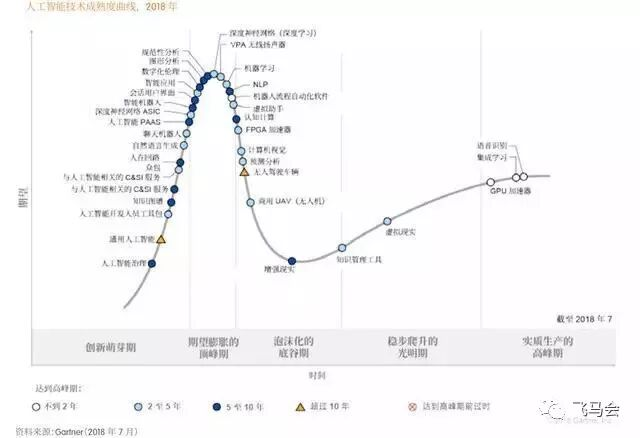2018 年新的人工智能技术成熟度曲线将由 Gartner 公司 67 位国际分析师参与定制编写，相较于 2017 年，2018 年新版本曲线将对更多技术的成熟度及预测做出更新，深度学习、自然语言处理等 30 个技术点进行了移动和调整。

2018 世界人工智能产业发展蓝皮书指出，人工智能仍处于早期采用阶段，许多企业也还处在正在考虑采用 AI 和规划 AI 的阶段，但仅有 4% 的被调研企业已经投资并部署了 AI 技术。在 Gartner 的 AI 技术成熟度曲线中，仍旧有许多技术拥堵在期望膨胀期，但若要过渡到生产实施阶段，仍旧非常困难。企业可以蓝皮书中勾勒的四种风格的的“卓越中心”为框架，推动 AI 的采用，而且其中的关键在于要选择适于业务风格和应用需求的方法。

1) 产业链地图版块

2) 产业分布地图版块

3) 产业研究机构与政策版块

1) 产业发展环境

2) 技术环境

3) 全球 AI 企业的情况

4) 全球投融资的情况

5) 产业发展情况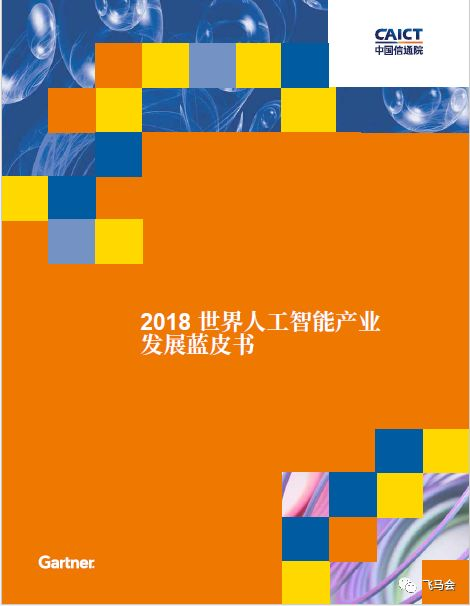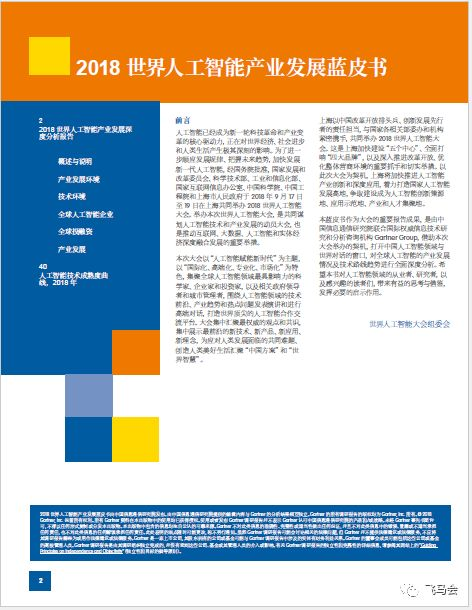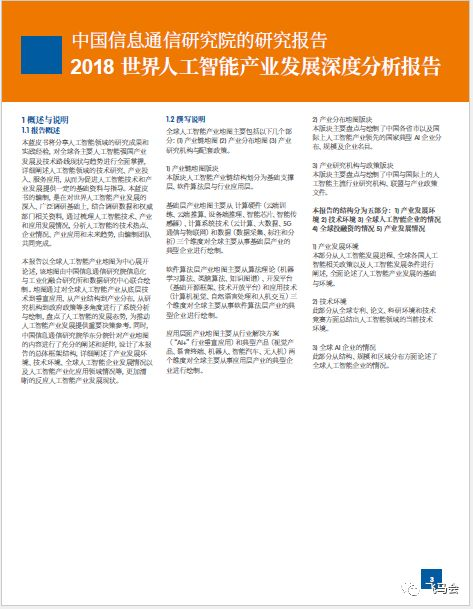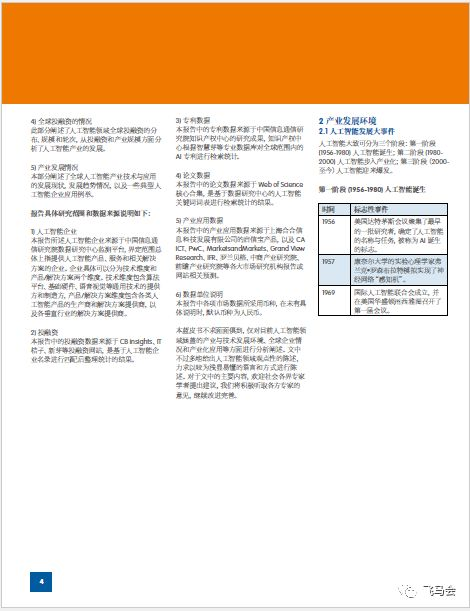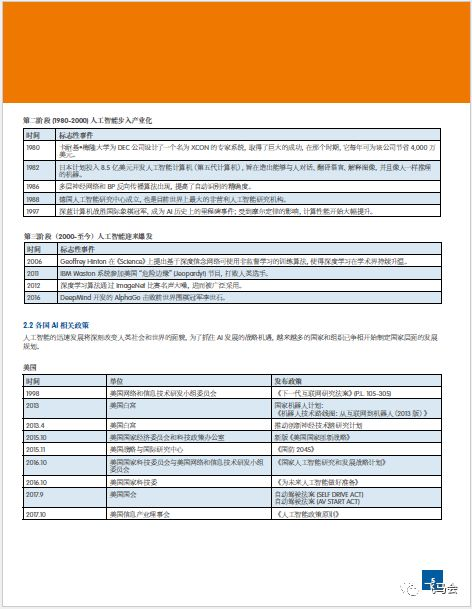《2018世界人工智能产业发展蓝皮书》